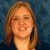## Setting up Microsoft Excel spreadsheet formulasTags:
Microsoft Excel formulas
Open source software
How do I set up spread sheet formulas. The help section that came with the software is useless to me as it is much too technical. Our children's charity nonprofit runs off one PC, and being able to use the spread sheet would be wonderful instead of making up documents adding on a calculator for hours and then putting it a word style document for our records.

Thanks. We'll let you know when a new response is added.

Try looking for some online Excel tutorials. The concepts are the same in most spreadsheet programs. The general functions are about the same but may have a slightly different name and that is where the online help would be useful in seeing what the function name is in one spreadsheet program vs another.

One online Excel tutorial resource I found is at MIStupid.com.

————————————

I think you will need to specify the “brand” or “name” of the spread sheet program, since you included an ‘excel formulas’ tag, but also an ‘open source applications for linux’ tag.

If the pc is running linux, it could be Star Office, or Open Office, which are pretty similar to Excel.

In general, to write a formula, you need to start with the equal sign (=), for example, to compute a sum for a range of cells (say from A3 to A7), you would write this formula:

=sum(A3:A7)

or you could write this:

=A3+A4+A5+A6

Usually you do not need to write the cell names, but select them with the mouse.

## Discuss This Question: 2 Replies

•